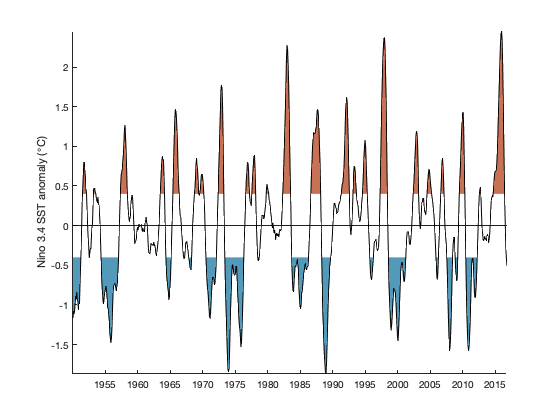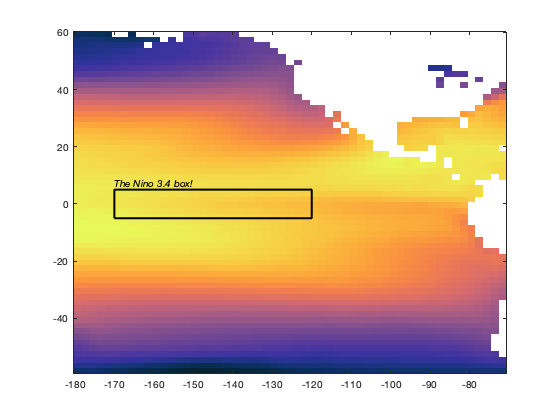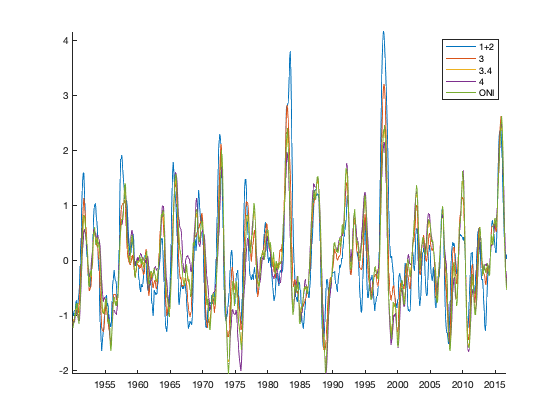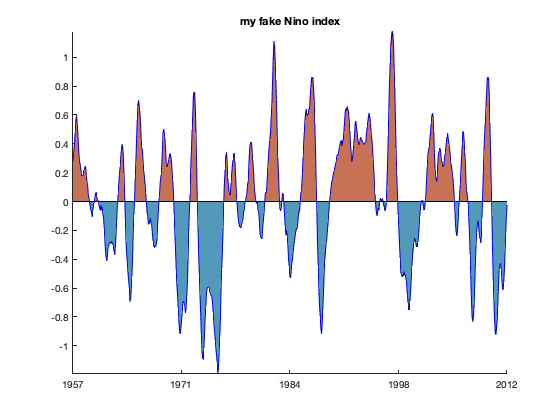# enso documentation

enso computes a version of the Nino 3.4 SST index.

Back to Climate Data Tools Contents

## Syntax

```idx = enso(sst,t)
idx = enso(sst,t,lat,lon)
idx = enso(sst,t,lat,lon,'region',NinoRegion)
idx = enso(...,'smoothing',months)```

## Description

idx = enso(sst,t) calculates El Nino Southern Oscillation index from a time series of sea surface temperatures sst and their corresponding times t. sst can be a vector of sea surface temperatures that have been averaged over a region of interest, or sst can be a 3D matrix whose third dimension correponds to times t. If sst is a 3D matrix, a time series is automatically generated by averaging all the grid cells in sst for each time step.

idx = enso(sst,t,lat,lon) calculates the Nino 3.4 index for 3D sst time series and corresponding grid coordinates lat,lon. Using this syntax, grid cells within the Nino 3.4 region are automatically determined and the Nino index is calculated from the area-averaged time series of ssts within that region.

idx = enso(sst,t,lat,lon,'region',NinoRegion) allows any of the following Nino regions, entered as string (in 'single quotes')

• '1+2'
• '3'
• '3.4' (default when lat and lon are specified)
• '4'
• 'ONI'

idx = enso(sst,t,mask) calculates the sst index using the unweighted mean of sst grid cells corresponding to true values in a 2D logical mask.

idx = enso(...,'smoothing',months) defines the moving average window in months. Default value is 5, following Trenberth 1997. The only exception is for the ONI region, which has a smoothing window of 3 months. To turn off averaging, set 'smoothing',false.

## Example 1: Automatic Nino 3.4

For this example, calculate the Nino 3.4 index using the monthly pacific_sst.mat dataset that comes with CDT. Start by loading the data, and then the enso function will want to know exactly what geo coordinates correspond to each grid cell in the sst dataset, so use meshgrid to get the lat,lon arrays into 2D grids we'll call Lat,Lon:

```load pacific_sst.mat

% Get 2D grids from lat,lon arrays:
[Lon,Lat] = meshgrid(lon,lat);
```

The simplest way to use the enso function is to enter the 3D sst data, the corresponding times t, and the grid cell coordinates, like this:

```idx = enso(sst,t,Lat,Lon);
```

## Plot it up real nice

With that, we can now plot the Nino 3.4 anomalies. We'll use the anomaly function with thresholds of +/- 0.4 degrees to identify El Nino and La Nina periods, respectively:

```figure
anomaly(t,idx,'thresh',[-0.4 0.4]);
axis tight
hline(0,'k') % places a horizontal line at 0
datetick('x','keeplimits')
ylabel 'Nino 3.4 SST anomaly (\circC)'
```## A bit of context

For a bit more context, and to understand what the enso function is doing, here's the mean sea surface temperature from the pacific_sst dataset, plotted with imagescn and using the cmocean thermal colormap:

```figure
imagescn(Lon,Lat,mean(sst,3))
cmocean thermal
```By default the enso function calculates the the Nino index using the lat,lon bounds of the Nino 3.4 box (5N-5S; 170W-120W). Here's the Nino 3.4 region on a map:

```% Define the Nino 3.4 box:
latv = [-5 -5 5 5 -5];
lonv = [-170 -120 -120 -170 -170];

% Plot the Nino 3.4 box:
hold on
plot(lonv,latv,'k-','linewidth',2)
text(-170,5,'The Nino 3.4 box!','vert','bottom','fontangle','italic')
```If you'd like to explore Nino indices in regions other than the default Nino 3.4 region, read on...

## Example 2: A manual approach

By default, the enso function calculates the area-weighted average sea surface temperature within the Nino 3.4 box, then uses deseason to remove seasonal cycles. The the mean is removed from the deseasoned time series to get the ENSO anomalies, and then smoothed using scatstat1 as a moving-average filter. The enso function allows you to override most of the steps of the Nino calculation or set them to your liking. Below are some examples of how to customize use of the enso function.

## Defining the Nino region

To use one of the predefined Nino regions other than the default '3.4', simply specify it like this:

```idx3 = enso(sst,t,Lat,Lon,'region','3');
```

Loop through all the predefined Nino regions to compare:

```% List the predefined regions:
regions = {'1+2','3','3.4','4','ONI'};

figure
hold on

% Loop through each region, calculate its Nino index, and plot:
for k = 1:length(regions)
tmp = enso(sst,t,Lat,Lon,'region',regions{k});
plot(t,tmp)
end
axis tight
datetick('x','keeplimits')
box off
legend(regions)
```Suppose you come up with a better region for characterizing El Nino and La Nina. It's the whole region from 20 S to 20 N, and from 170 W to 110 W, but only where grid cell latitudes and longitudes minus half a degree are divisible by 3. Use geomask to determine which grid cells lie within that region and use mod to figure out which grid cells (minus 0.5 degrees) are divisble by 3. Here's what the mask looks like:

```mask = geomask(Lat,Lon,[-20 20],[-170 -110]) ...
& mod(Lat-0.5,3)==0 & mod(Lon-0.5,3)==0;

figure
borders
```If you'd like to get an unweighted Nino index for the region shown as yellow cells in the mask above, do this:

```idx = enso(sst,t,mask);

figure
anomaly(t,idx)
axis tight
datetick('x','keepticks')
title 'my fake Nino index'
```To account for the fact that the grid cells in your mask are not all equal in surface area on the globe use cdtarea to get the area of each grid cell, then use local to get the area-weighted time series of sea surface temperatures within your mask:

```% Get the area of each grid cell:
A = cdtarea(Lat,Lon);

% Get the area-weighted time series in the Nino 3.4 box:
```

Now sst_myregion is an 802x1 array of area-averaged sea surface temperatures in your mask, and and it can be entered directly into the enso function to convert it to an index:

```idx_myregion = enso(sst_myregion,t);
```

Plotting the area-weighted index on top of the unweighted anomaly plot, you will see that they are quite similar. Here's the weighted version plotted as a blue line on top of the anomaly plot we created above:

```hold on
plot(t,idx_myregion,'b')
```Whether or not we account for grid cell dimensions when calculating the mean sst within a mask, it doesn't seem to matter much here. That's because these grid cells are all within a relatively narrow range of latitudes close to the equator, where grid cells are all about the same size.

## Example 3: Recreating Trenberth 1997

In this example, we'll recreate Figure 1 from Trenberth's 1997 classic paper, The Definition of El Nino (Trenberth, 1997), which depicts the ENSO index calculated from Pacific sea surface temperatures.

We'll use the same pacific_sst dataset from the examples above. First, calculate the Nino 3 and Nino 3.4 indices:

```idx_3 = enso(sst,t,Lat,Lon,'region','3');
idx_34 = enso(sst,t,Lat,Lon,'region','3.4');
```

Now recreate both subplots of Trenberth's Figure 1, using subsubplot:

```figure
subsubplot(2,1,1)
anomaly(t,idx_3,'top',rgb('charcoal'),...
'bottom',rgb('gray'),'thresh',[-0.5 0.5]);

hline([-0.5 0.5],'k:')
hline(0,'k','linewidth',1)

xlim(datenum([1950 1997.5],1,1))
ylim([-2 3])
ntitle(' Nino 3 Region (Threshold = 0.5\circC) ','location','nw')

subsubplot(2,1,2)
anomaly(t,idx_34,'top',rgb('charcoal'),...
'bottom',rgb('gray'),'thresh',[-0.4 0.4]);

hline([-0.4 0.4],'k:')
hline(0,'k','linewidth',1)

xlim(datenum([1950 1997.5],1,1))
ylim([-2 3])
ntitle(' Nino 3.4 Region (Threshold = 0.4\circC) ','location','nw')

datetick('x','keeplimits')
xlabel('Year')
```## References

Trenberth, Kevin E. "The Definition of El Nino." Bulletin of the American Meteorological Society 78.12 (1997): 2771-2778. https://doi.org/10.1175/1520-0477(1997)078%3C2771:TDOENO%3E2.0.CO;2

## Author Info

The enso function and supporting documentation were written by Thirumalai <http://www.kaustubh.info> and Chad A. Greene for the Climate Data Toolbox for Matlab, 2019.